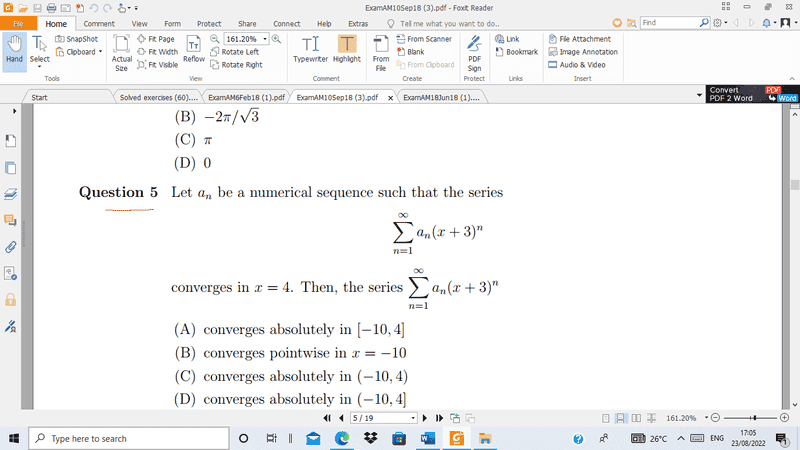# Convergence of a series

• Amaelle
Any hint?thank you!Set ##y = x +3##. The radius of convergence wrt ##y## must be an interval centred on ##y =0##.Set ##y = x +3##. The radius of convergence wrt ##y## must be an interval centred on ##y =0##.f

#### Amaelle

Homework Statement
look at the image
Relevant Equations
Set of convergence
GreetingsAccording to my understanding: if x converges in 4 means that the series converges -1<x+3<7 but the solution says C
Any hint?
thank you!

Set ##y = x +3##. The radius of convergence wrt ##y## must be an interval centred on ##y =0##.

Set ##y = x +3##. The radius of convergence wrt ##y## must be an interval centred on ##y =0##.
thank you this is what I have done
y=x+3
x conveges in 4 then
y converges in ]-1,7[

thank you this is what I have done
y=x+3
x conveges in 4 then
y converges in ]-1,7[
The radius of convergence is an interval centred on ##0##. It cannot be ##[-1,7]##.

The radius of convergence is an interval centred on ##0##. It cannot be ##[-1,7]##.
the exercice ask about the set of convergence and not the radius and as you can see we have y=x+3 y is centred in 0 not x

y is centred in 0 not x
That's not what you have. You have ##y## centred on ##3##:
y converges in ]-1,7[

The width of the interval of convergence is two times the radius of convergence.

The phrase "converges in x=4" is strange. It should be "converges AT x=4". That tells you that with x=4, we are in or exactly at the boundary of convergence at x=4. So (x+3)=7 is inside or at the radius of convergence of the power series.
So, what can you say about the region of convergence? Be careful about where the convergence is absolute versus other convergence. That makes a big difference exactly at the endpoints of the region of convergence.

thank you this is what I have done
y=x+3
x conveges in 4 then
y converges in ]-1,7[
Neither x nor y "converges in" some point or some interval. It's the series that converges, not values of x or y.
The phrase "converges in x=4" is strange. It should be "converges AT x=4".
That phrasing bothers me as well. I agree that it should be "converges AT x =4" or wherever.

Homework Statement:: look at the image
Relevant Equations:: Set of convergence

Greetings
View attachment 313163
According to my understanding: if x converges in 4 means that the series converges -1<x+3<7
This sounds wrong. Are you thinking that "converges in x=4" means that the radius of convergence is 4? That is not how I would interpret it. I would guess that the phrase was bad and they meant "converges at x=4", which makes it converge for (x+3)=7. So the radius of convergence is 7. That will change your endpoints of the region of convergence.